Practice Test: Polynomials

# Practice Test: Polynomials - Class 10

Test Description

## 15 Questions MCQ Test Mathematics (Maths) Class 10 - Practice Test: Polynomials

Practice Test: Polynomials for Class 10 2023 is part of Mathematics (Maths) Class 10 preparation. The Practice Test: Polynomials questions and answers have been prepared according to the Class 10 exam syllabus.The Practice Test: Polynomials MCQs are made for Class 10 2023 Exam. Find important definitions, questions, notes, meanings, examples, exercises, MCQs and online tests for Practice Test: Polynomials below.
Solutions of Practice Test: Polynomials questions in English are available as part of our Mathematics (Maths) Class 10 for Class 10 & Practice Test: Polynomials solutions in Hindi for Mathematics (Maths) Class 10 course. Download more important topics, notes, lectures and mock test series for Class 10 Exam by signing up for free. Attempt Practice Test: Polynomials | 15 questions in 20 minutes | Mock test for Class 10 preparation | Free important questions MCQ to study Mathematics (Maths) Class 10 for Class 10 Exam | Download free PDF with solutions
 1 Crore+ students have signed up on EduRev. Have you?
Practice Test: Polynomials - Question 1

### The zeroes of the quadratic polynomial x2 + ax + b, a,b > 0 are

Detailed Solution for Practice Test: Polynomials - Question 1

p(x)=x2+ax+b

Let one of the zero of the polynomial is a then the other zero will be −a.

Sum of zeros=a+(−a)= −a / 1

=>0= −a/1

=>a=0

and product of the zeros =a(−a)=b/1

=>−a2=b

=>b=−a2

=>b has to be negative.

Practice Test: Polynomials - Question 2

### If p(x) = ax2 + bx + c and a + b + c = 0, then one zero is

Detailed Solution for Practice Test: Polynomials - Question 2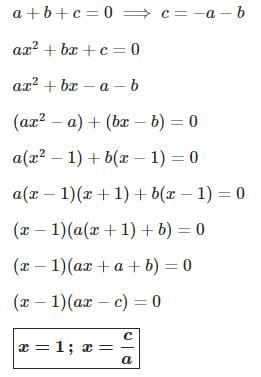Practice Test: Polynomials - Question 3

### Given that one of the zeroes of the cubic polynomial ax3 + bx2 + cx + d is zero, the product of the other two zeros is

Detailed Solution for Practice Test: Polynomials - Question 3

Let p(x) =ax3 + bx2 + cx + d

Given that, one of the zeroes of the cubic polynomial p(x) is zero.

Let α, β and γ are the zeroes of cubic polynomial p(x), where α = 0.

We know that,

Step-by-step explanation:

sum of two zeros at a time = c/a

∴αβ + βy + yα = c/a

∴0×β + βy + y×0 = c/a

βy = c/a

hence, product of 2 zeros = c/a

Practice Test: Polynomials - Question 4

If p(x) = ax2 + bx + c and a + c = b, then one of the zeroes is

Detailed Solution for Practice Test: Polynomials - Question 4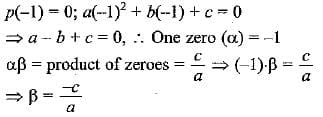Practice Test: Polynomials - Question 5

If one of the zeros of the quadratic polynomial  (k - 1) x2 + k x + 1 is - 3, then the value of k is

Practice Test: Polynomials - Question 6

The number of polynomials haying zeroes as -2 and 5 is

Detailed Solution for Practice Test: Polynomials - Question 6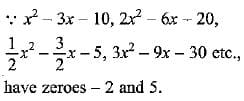Practice Test: Polynomials - Question 7

If one of the zeros  of the cubic polynomial x3 + ax2 + bx + c is - 1, then the product of the other two zeroes is

Detailed Solution for Practice Test: Polynomials - Question 7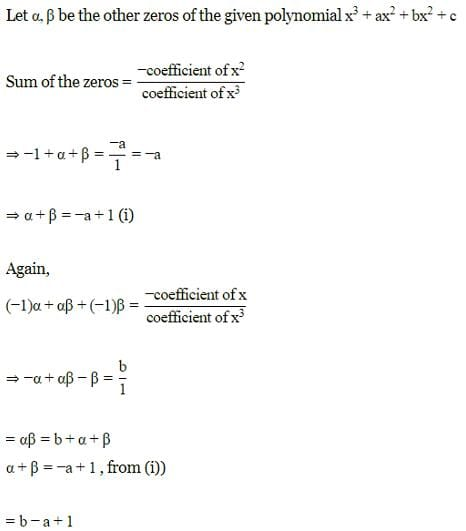Practice Test: Polynomials - Question 8

Given that one of the zeroes of the cubic polynomial ax3 + bx2 + cx + d is zero, the product of the other two zeroes is

Detailed Solution for Practice Test: Polynomials - Question 8

Let p(x) =ax3 + bx2 + cx + d
Given that, one of the zeroes of the cubic polynomial p(x) is zero.
Let α, β and γ are the zeroes of cubic polynomial p(x), where a = 0.
We know that,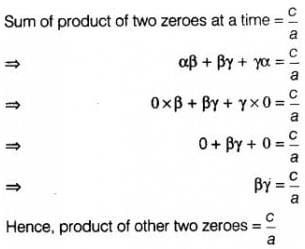Practice Test: Polynomials - Question 9

Given that two of the zeros of the cubic polynomial ax3 + bx2 + cx + d are 0, the value of c is

Practice Test: Polynomials - Question 10

If 4x2 - 6x - m is divisible by x - 3, the value of m is exact divisor of

Detailed Solution for Practice Test: Polynomials - Question 10

Here p(3) = 0
⇒ 4(3)2 - 6 x 3 - m = 0
⇒ 36 - 18 - m = 0 ⇒ m =18
∴ Value of m is exactly divisible by 9.

Practice Test: Polynomials - Question 11

A quadratic polynomial, whose zeros are 5 and - 8 is

Practice Test: Polynomials - Question 12

Which one of the following statements is correct

Detailed Solution for Practice Test: Polynomials - Question 12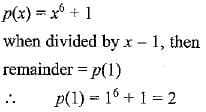Practice Test: Polynomials - Question 13

The zeros of the quadratic polynomial x2 + kx + k, k ≠ 0

Practice Test: Polynomials - Question 14

Consider the following statements
(i) x - 2 is a factor of x3 - 3x2 + 4x - 4.
(ii) x + 1 is a factor of 2x3 + 4x + 6
(iii) x - 1 is a factor of x5 + x4 - x3 + x2 - x + 1

In these statements

Detailed Solution for Practice Test: Polynomials - Question 14

x - 2 is a factor of x3 - 3x2 + 4x - 4
∵ remainder is zero
Similarly x + 1 is a factor of 2x3 + 4x + 6
but x - 1 is not a factor of x5 + x4 - x3 + x2 - x + 1
∵ remainder is not zero
∴ Statements 1 and 2 are correct.

Practice Test: Polynomials - Question 15

The degree of the remainder r(x) when p (x) = bx3 + cx + d is divided by a polynomial of degree 4 is ​

## Mathematics (Maths) Class 10

115 videos|478 docs|129 tests
Information about Practice Test: Polynomials Page
In this test you can find the Exam questions for Practice Test: Polynomials solved & explained in the simplest way possible. Besides giving Questions and answers for Practice Test: Polynomials, EduRev gives you an ample number of Online tests for practice

## Mathematics (Maths) Class 10

115 videos|478 docs|129 tests